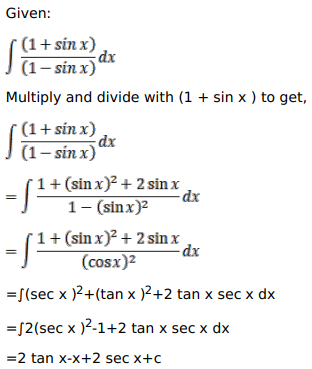# Mark against the correct answer in each of the following:

Question:

Mark $(\sqrt{)}$ against the correct answer in each of the following:

$\int \frac{(1+\sin x)}{(1-\sin x)} d x=?$

A. $2 \tan x+2 \sec x+x+C$

B. $2 \tan x+2 \sec x-x+C$

C. $\tan x+\sec x-x+C$

D. None of these

Solution: